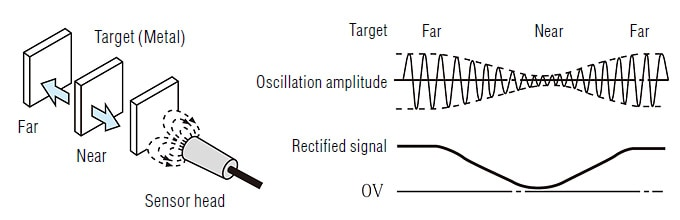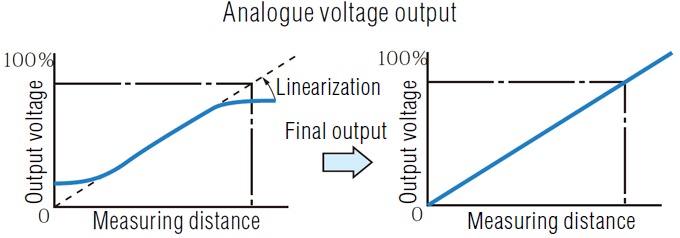# Detection based on “Changes in Eddy Current”What is an inductive displacement sensor?

“Inductive displacement sensors” not only detect the presence of a target but also measure the distance to a target.

### EX-V, EX-200 and AS Series

As the target approaches the sensor head, the eddy current loss increases and oscillation amplitude becomes smaller accordingly. This oscillation amplitude is rectified to obtain DC voltage variations.The rectified signal and distance have an approximate proportional relationship. The linearisation circuit corrects the linearity to obtain a linear output that is proportional to the distance.### EX-500 Series (All metal type)

As a target approaches the sensor head, the oscillation amplitude becomes smaller and the phase difference from the reference waveform becomes larger. By detecting changes in the amplitude and phase, the sensor can obtain a value approximately proportional to the distance. Then, a high-accuracy linearisation processing corrects the value digitally based on the target material, to obtain a linear output that is proportional to the distance.The EX-500 Series detects both amplitude and phase difference to enable detection of nonferrous metals such as copper and aluminium so that it can be an all metal supporting sensor. When only the magnitude of the amplitude is detected, it is hard to determine whether it is changing due to the change in the material or due to the distance to the target. Therefore, the sensor detects the change in the phase as well to verify the change in the material.

INDEX